## 7.5. 接口值

var w io.Writer
w = os.Stdout
w = new(bytes.Buffer)
w = nil


var w io.Writer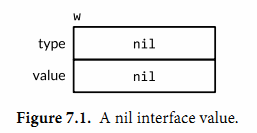w.Write([]byte("hello")) // panic: nil pointer dereference


w = os.Stdout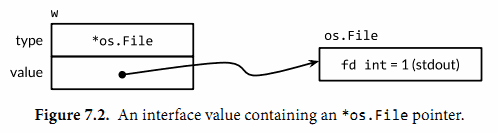w.Write([]byte("hello")) // "hello"


os.Stdout.Write([]byte("hello")) // "hello"


w = new(bytes.Buffer)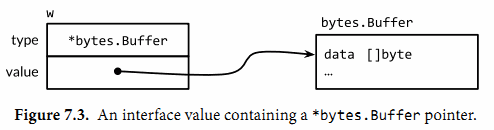Write方法的調用也使用了和之前一樣的機製：

w.Write([]byte("hello")) // writes "hello" to the bytes.Buffers


w = nil


var x interface{} = time.Now()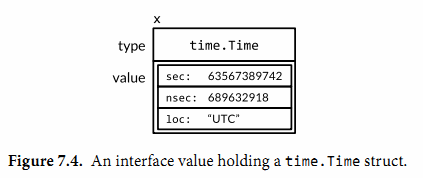var x interface{} = []int{1, 2, 3}
fmt.Println(x == x) // panic: comparing uncomparable type []int


var w io.Writer
fmt.Printf("%T\n", w) // "<nil>"
w = os.Stdout
fmt.Printf("%T\n", w) // "*os.File"
w = new(bytes.Buffer)
fmt.Printf("%T\n", w) // "*bytes.Buffer"


### 7.5.1. 警告：一個包含nil指針的接口不是nil接口

const debug = true

func main() {
var buf *bytes.Buffer
if debug {
buf = new(bytes.Buffer) // enable collection of output
}
f(buf) // NOTE: subtly incorrect!
if debug {
// ...use buf...
}
}

// If out is non-nil, output will be written to it.
func f(out io.Writer) {
// ...do something...
if out != nil {
out.Write([]byte("done!\n"))
}
}


if out != nil {
out.Write([]byte("done!\n")) // panic: nil pointer dereference
}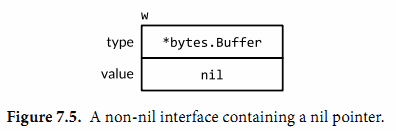var buf io.Writer
if debug {
buf = new(bytes.Buffer) // enable collection of output
}
f(buf) // OK